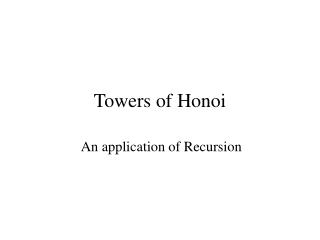# Towers of Honoi - PowerPoint PPT PresentationDownload PresentationTowers of Honoi

Towers of HonoiDownload Presentation## Towers of Honoi

- - - - - - - - - - - - - - - - - - - - - - - - - - - E N D - - - - - - - - - - - - - - - - - - - - - - - - - - -
##### Presentation Transcript

1. Towers of Honoi An application of Recursion

2. Towers of Hanoi Problem: Invented by French mathematician Lucas in 1880s. Original problem set in India in a holy place called Benares. There are 3 diamond needles fixed on a brass plate. One needle contains 64 pure gold disks. Largest resting on the brass plate , other disks of decreasing diameters. Called tower of Brahma. Priests are supposed to transfer the disks from one needle to the other such that at no time a disk of larger diameter should sit on a disk of smaller diameter. Only one disk can be moved at a time. Later setting shifted to Honoi, but the puzzle and legend remain the same. How much time would it take? Estimate…..

3. Finding a Recursive strategy: Any tower with more than one disk must be moved in pieces. If there is just one disk, then move it. It must be possible to break the problem into simpler subproblems of same form.

4. 3 Disks start temp finish

5. Irrespective of number of disks, the following steps need to be carried out: The bottom most disk needs to be moved to finish tower. So the disks above it must be removed first in step 1. In step 2,the bottom most disk can be moved. In step 3, the disks above it must be replaced in proper position. Now let us consider the problem of 3 disks.

6. start temp finish start Step 1: Move 2 disks from start to temp using finish Tower. To understand the recursive routine, let us assume that we know how to solve 2 disk problem, and go for the next step.

7. start temp finish Step 2: Move the (remaining) single disk from start to finish. This does not involve recursion, and can be carried out without using temp tower.

8. start temp finish Step 3: Now we are at the last step of the recursive routine. Move the 2 disks from temp tower to finish tower using the start tower ( recursively).

9. start temp finish This will solve the 3 disk problem. Now let us see how the complete sequence can be worked out and find out how many steps are going to be actually needed.

10. The Complete sequence for 3 Disks start temp finish

11. start temp finish start temp finish start temp finish start temp finish start temp finish start 1 2 3 4

12. start temp finish start temp finish start temp finish 5 6 7

13. void movetower ( n, start, finish, temp) { if ( n==1) { movesingle ( start, finish); } else { movetower ( n-1, start, temp, finish); movesingle ( start, finish); movetower ( n-1, temp, finish, start); } T(n) = 2 T(n-1) + 1

14. How many steps? disks No. of steps 1 1 2 3 ( 1 + 1 + 1 ) 3 7 ( 3 + 1 + 3 ) 4 15 (7 + 1 + 7 ) n use recurrence relation Recurrence relation: T(n) = 2 T(n – 1 ) + 1

15. T(n) = 2 T(n – 1 ) + 1 = 2 [ 2 T(n – 2) + 1] + 1 = 4 T(n – 2) + 2 + 1 = 4 [ 2 T(n – 3) + 1] + 3 = 8 T(n – 3) + 7 = 23 T(n – 3) + 23 – 1 =2k T(n – k) + 2k – 1 Now let n – k = 1 = 2n-1 T(1) + 2n-1 – 1 as time for 1 disk: T(1) = 1 = 2. 2n-1 – 1 = 2n – 1 = O( 2n )